# wavedec

1-D wavelet decomposition

## Syntax

``[c,l] = wavedec(x,n,wname)``
``[c,l] = wavedec(x,n,LoD,HiD)``

## Description

example

````[c,l] = wavedec(x,n,wname)` returns the wavelet decomposition of the 1-D signal `x` at level `n` using the wavelet `wname`. The output decomposition structure consists of the wavelet decomposition vector `c` and the bookkeeping vector `l`, which contains the number of coefficients by level. NoteFor `gpuArray` inputs, the supported modes are `'symh'` (`'sym'`) and `'per'`. If the input is a `gpuArray`, the discrete wavelet transform extension mode used by `wavedec` defaults to `'symh'` unless the current extension mode is `'per'`. See the example Multilevel Discrete Wavelet Transform on a GPU. ```
````[c,l] = wavedec(x,n,LoD,HiD)` returns the wavelet decomposition using the specified lowpass and highpass wavelet decomposition filters `LoD` and `HiD`, respectively.```

## Examples

collapse all

Load and plot a one-dimensional signal.

```load sumsin plot(sumsin) title('Signal')```Perform a 3-level wavelet decomposition of the signal using the order 2 Daubechies wavelet. Extract the coarse scale approximation coefficients and the detail coefficients from the decomposition.

```[c,l] = wavedec(sumsin,3,'db2'); approx = appcoef(c,l,'db2'); [cd1,cd2,cd3] = detcoef(c,l,[1 2 3]);```

Plot the coefficients.

```subplot(4,1,1) plot(approx) title('Approximation Coefficients') subplot(4,1,2) plot(cd3) title('Level 3 Detail Coefficients') subplot(4,1,3) plot(cd2) title('Level 2 Detail Coefficients') subplot(4,1,4) plot(cd1) title('Level 1 Detail Coefficients')```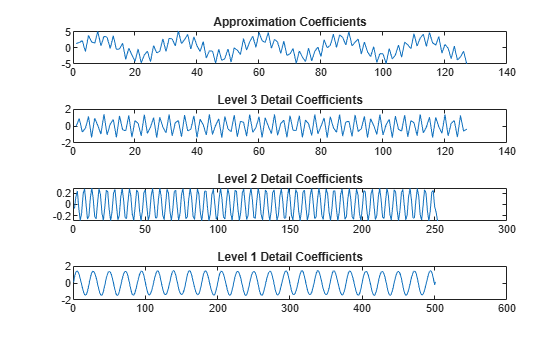Refer to GPU Support by Release (Parallel Computing Toolbox) to see what GPUs are supported.

Load the noisy Doppler signal. Put the signal on the GPU using `gpuArray`. Save the current extension mode.

```load noisdopp noisdoppg = gpuArray(noisdopp); origMode = dwtmode('status','nodisp');```

Use `dwtmode` to change the extension mode to zero-padding. Obtain the three-level DWT of the signal on the GPU using the `db4` wavelet.

```dwtmode('zpd','nodisp') [c,l] = wavedec(noisdoppg,3,'db4');```

The current extension mode `zpd` is not supported for `gpuArray` input. Therefore, the DWT is instead performed using the `sym` extension mode. To confirm this, set the extension mode to `sym` and take DWT of `noisdoppg`, then compare with the previous result.

```dwtmode('sym','nodisp') [csym,lsym] = wavedec(noisdoppg,3,'db4'); [max(abs(c-csym)) max(abs(l-lsym))]```
```ans = 0 0 ```

Set the current extension mode to `per` and obtain the three-level DWT of `noisdopp`. The extension mode `per` is supported for `gpuArray` input. Confirm the result is different from the `sym` results.

```dwtmode('per','nodisp') [cper,lper] = wavedec(noisdoppg,3,'db4'); [length(csym) ; length(cper)]```
```ans = 2×1 1044 1024 ```
`[lsym ; lper]`
```ans = 2×5 134 134 261 515 1024 128 128 256 512 1024 ```

Restore the extension mode to the original setting.

`dwtmode(origMode,'nodisp')`

## Input Arguments

collapse all

Input signal, specified as a vector.

Data Types: `single` | `double`
Complex Number Support: Yes

Level of decomposition, specified as a positive integer. `wavedec` does not enforce a maximum level restriction. Use `wmaxlev` to ensure that the wavelet coefficients are free from boundary effects. If boundary effects are not a concern in your application, a good rule is to set `n` less than or equal to `fix(log2(length(x)))`.

Data Types: `single` | `double`

Analyzing wavelet, specified as a character vector or string scalar.

Note

`wavedec` supports only Type 1 (orthogonal) or Type 2 (biorthogonal) wavelets. See `wfilters` for a list of orthogonal and biorthogonal wavelets.

Wavelet decomposition filters, specified as a pair of even-length real-valued vectors. `LoD` is the lowpass decomposition filter, and `HiD` is the highpass decomposition filter. The lengths of `LoD` and `HiD` must be equal. See `wfilters` for additional information.

Data Types: `single` | `double`

## Output Arguments

collapse all

Wavelet decomposition vector, returned as a vector. The bookkeeping vector `l` contains the number of coefficients by level. The decomposition vector and bookkeeping vector are organized as in this level-3 decomposition diagram.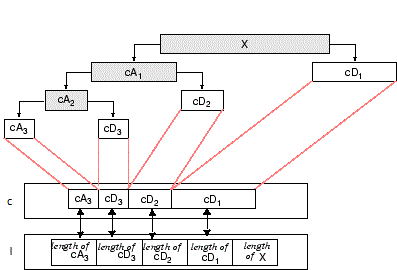Data Types: `single` | `double`

Bookkeeping vector, returned as a vector of positive integers. The bookkeeping vector is used to parse the coefficients in the wavelet decomposition vector `c` by level.

Data Types: `single` | `double`

## Algorithms

Given a signal s of length N, the DWT consists of at most log2 N steps. Starting from s, the first step produces two sets of coefficients: approximation coefficients cA1 and detail coefficients cD1. Convolving s with the lowpass filter `LoD` and the highpass filter `HiD`, followed by dyadic decimation (downsampling), results in the approximation and detail coefficients respectively.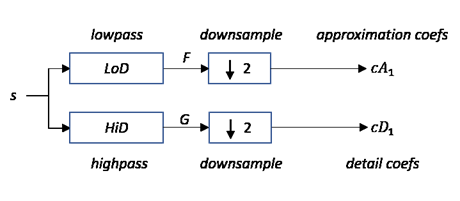where

•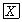— Convolve with filter X

• $\begin{array}{||}\hline ↓2\\ \hline\end{array}$ — Downsample (keep the even-indexed elements)

The length of each filter is equal to 2n. If N = length(s), the signals F and G are of length N + 2n −1 and the coefficients cA1 and cD1 are of length

floor$\left(\frac{N-1}{2}\right)+n$.

The next step splits the approximation coefficients cA1 in two parts using the same scheme, replacing s by cA1, and producing cA2 and cD2, and so on.The wavelet decomposition of the signal s analyzed at level j has the following structure: [cAj, cDj, ..., cD1].

This structure contains, for j = 3, the terminal nodes of the following tree: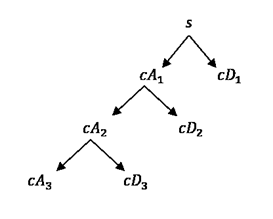Daubechies, I. Ten Lectures on Wavelets, CBMS-NSF Regional Conference Series in Applied Mathematics. Philadelphia, PA: SIAM Ed, 1992.

 Mallat, S. G. “A Theory for Multiresolution Signal Decomposition: The Wavelet Representation,” IEEE Transactions on Pattern Analysis and Machine Intelligence. Vol. 11, Issue 7, July 1989, pp. 674–693.

 Meyer, Y. Wavelets and Operators. Translated by D. H. Salinger. Cambridge, UK: Cambridge University Press, 1995.

## SupportGet trial now